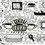# Perfect Powers

How many $3$ digit numbers $N$ can be expressed as $\overline{aba} = p^q$, where $p$ and $q$ are positive integers and $q>1$

$A$ $request$ : Please explain your steps a bit if you use Modular Arithmetic because I ain't much used to it.Note by Abhimanyu Swami
6 years, 10 months ago

This discussion board is a place to discuss our Daily Challenges and the math and science related to those challenges. Explanations are more than just a solution — they should explain the steps and thinking strategies that you used to obtain the solution. Comments should further the discussion of math and science.

When posting on Brilliant:

• Use the emojis to react to an explanation, whether you're congratulating a job well done , or just really confused .
• Ask specific questions about the challenge or the steps in somebody's explanation. Well-posed questions can add a lot to the discussion, but posting "I don't understand!" doesn't help anyone.
• Try to contribute something new to the discussion, whether it is an extension, generalization or other idea related to the challenge.

MarkdownAppears as
*italics* or _italics_ italics
**bold** or __bold__ bold
- bulleted- list
• bulleted
• list
1. numbered2. list
1. numbered
2. list
Note: you must add a full line of space before and after lists for them to show up correctly
paragraph 1paragraph 2

paragraph 1

paragraph 2

[example link](https://brilliant.org)example link
> This is a quote
This is a quote
    # I indented these lines
# 4 spaces, and now they show
# up as a code block.

print "hello world"
# I indented these lines
# 4 spaces, and now they show
# up as a code block.

print "hello world"
MathAppears as
Remember to wrap math in $$ ... $$ or $ ... $ to ensure proper formatting.
2 \times 3 $2 \times 3$
2^{34} $2^{34}$
a_{i-1} $a_{i-1}$
\frac{2}{3} $\frac{2}{3}$
\sqrt{2} $\sqrt{2}$
\sum_{i=1}^3 $\sum_{i=1}^3$
\sin \theta $\sin \theta$
\boxed{123} $\boxed{123}$

Sort by:

Again I believe there is no better choice than a case-by-case analysis. I rephrase the problem to find the number of 3-digit palindrome perfect power. First note that no power of 2 is a 3-digit palindrome. Since $3^7>1000$, we just need to check until $q=6$. Furthermore, if $q$ is even, $p^q$ is a perfect square and we may assume $q=2$. Now we consider $q=5,3,2$.

Case 1: $q=5$

$4^5>1000$, and $3^5$is not a palindrome.

Case 2: $q=3$

Just manually check for $1\leq p\leq 9$, and we find only $7^3=343$ is a palindrome.

Case 3: $q=2$

Recall that the quadratic residues modulo $10$ are $1,4,9,6,5$. Substitute $a=1,4,9,6,5$ and check to find a $b$ such that $\overline{aba}$ is a square. One arrives at $11^2=121, 22^2=484, 26^2=676$.

Therefore we conclude there are 4 3-digit palindrome perfect powers.

- 6 years, 10 months ago

Thanks again.

- 6 years, 10 months ago

You're welcome.

- 6 years, 10 months ago

The answer is 4. (7^3 = 343, 11^2 = 121, 22^2 = 484, 26^2 = 676) *Brute force. (I'll try to prove this later.)

- 6 years, 10 months ago